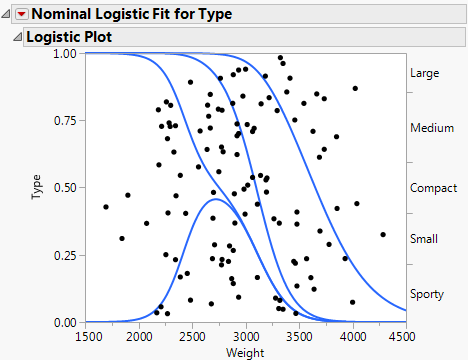Fitting Linear Models > Logistic Regression Models
Publication date: 07/30/2020

# Logistic Regression Models

##### Fit Regression Models for Nominal or Ordinal Responses

When your response variable has discrete values, you can use the Fit Model platform to fit a logistic regression model. The Fit Model platform provides two personalities for fitting logistic regression models. The personality that you use depends on the modeling type (Nominal or Ordinal) of your response column.

For nominal response variables, the Nominal Logistic personality fits a linear model to a multi-level logistic response function.

For ordinal response variables, the Ordinal Logistic personality fits the cumulative response probabilities to the logistic distribution function of a linear model.

Both personalities provide likelihood ratio tests for the model, a confusion matrix, and ROC and lift curves. When the response is binary, the Nominal Logistic personality also provides odds ratios (with corresponding confidence intervals).

Figure 11.1 Logistic Plot for a Nominal Logistic Regression Model